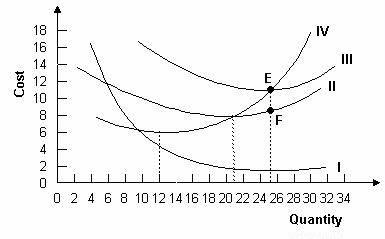### CFA Practice Question

There are 539 practice questions for this study session.

### CFA Practice Question

Refer to the graph below. Why does the distance between curves II and III get smaller?A. Marginal cost is increasing.
B. Average variable cost is increasing.
C. Average fixed cost is declining.

Average total cost is the sum of average variable cost and average fixed cost. Since average fixed cost declines as output increases, the average total and average variable cost curves must converge.

User Comment
tinku What do these curves represent?
evica AVC & ATC
cbb1 I is AFC (Average Fixed Cost)
II is AVC (Average Variable Cost)
III is ATC (Average Total Cost)
IV is MC (Marginal Cost)
rana1970 very good cbb1, would you like to share some other concepts with me?
Mariecfa The vertical distance between the ATC and AVC curves are equal to AFC. This is indicated at where points E and F distance from each other are and where Quantity is approximately 25.
schweitzdm I use "MTVF" to remember the order of these curves from top to bottom.
akbhagat96 Hi everyone, I think correct answer is B. Curve I is average fixed cost curve and that is almost parallel, that means AFC is not declining at point E/F. AVC curve will move upward and distance will decrease due to increase in AVC not decrease in ATC. Pls correct me if I am wrong
Hermalia @akbhagat96 the answer is C. The question does not refer to points E or F so don't need to worry about that. Compare this graph to the last one in the notes and you would see why the answer is C. Because while AVC is increasing that is not the reason AVC and ATV curves are getting closer together.
Huricane74 @akbhagat96, if you look at the graph, it looks like the average variable cost is increasing and the average fixed cost is almost constant.

Unfortunately, the graph is an illusion that is really used for illustration purposes.

As a result of the way the graph is drawn, it look like curve II and curve III are getting closer together because average variable cost is increasing faster than total cost is increasing.

In a manufacturing environment, your average variable cost tends to be constant. For example, in the auto industry, your average variable cost may be \$15,000 per car for a car that costs \$30,000.

However, as your production increases from a few thousand cars to a few million, your average fixed cost declines significantly.

Think of Tesla. They need to get their production of the Model 3 up, or they are going to incur significant loses because the fixed costs are already sunk and need to be allocated on a per vehicle bases. Suppose Tesla makes 5000 cars per year and their fixed costs are a half billion. The average fixed cost in this situation is \$100,000 per car.

However, if production increases to 50,000 cars, the average fixed cost is \$10,000 per car.

This is way the answer is C. This is why it is important to do the math associated with each problem. Only then can you completely understand and apply the concepts. Otherwise you are just memorizing answers.